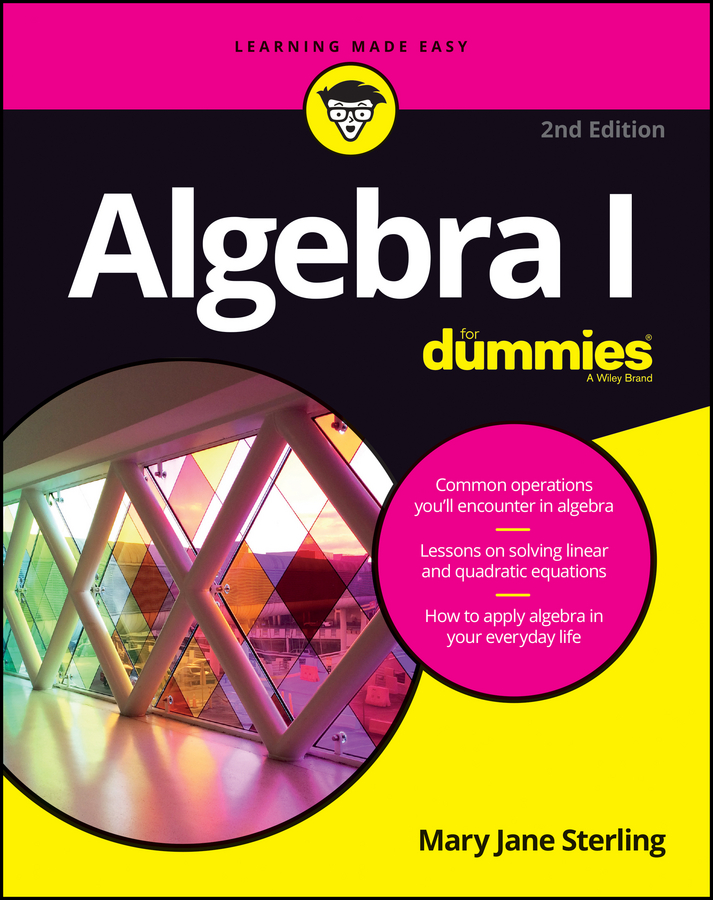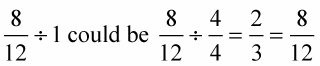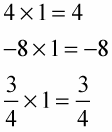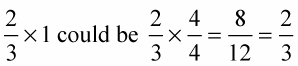##### Algebra I For DummiesIt’s always nice when you can reduce a fraction to make it more user-friendly. The fraction 3/4 is much nicer than 447/596. Sometimes, though, the fraction just doesn’t want to cooperate. In these cases, you still have options: you can round up or down, and then you can multiply or divide by one.

• Rounding up or down

Try reducing 25/36 into its lowest terms. Twenty-five can be divided by 5 or 25, and 36 can be divided by 2, 3, 4, 6, 9, 12, 18, and 36, but none of these match with 5 or 25. Even though 25 and 36 are not prime numbers, they have no common factor. The fraction cannot be reduced.

In cases where the fraction won’t reduce to lower terms, you can just leave well enough alone, or, if you’re so inclined, round the numerator or denominator up or down to make it reducible. For example, 301/498 doesn’t reduce. But, if you round the numerator down to 300 and the denominator up to 500, you get an approximate fraction 300/500 that reduces to 3/5. In this case, the rounding doesn’t change the value by much. You just have to use your judgment.

• Dividing by one

Any number divided by one equals that number: For any real number n, n 1 = n. So, knowing this allows you to change how a fraction looks without changing its value. See how it works in the following example?You do the same thing on the top and bottom of the fraction, so you really just divided by one, which doesn’t change the value — just how it looks.

• Multiplying by one

Any number multiplied by one equals that number: For any real number n, n 1 = n. Like division, you can multiply by one and change how a fraction looks without changing its value. That is,In the case of fractions, instead of actually using one, a fraction equal to one is used.Using the fractional value for the number one allows you to change how fractions look without changing their value.